## Acid and Base Ionization Constants

### Learning Objectives

• Define a strong acid.
• List some strong acids.
• Define a weak acid.
• List some weak acids.
• Write the expression for the acid ionization constant.
• Define strong and weak bases.
• Give examples of strong and weak bases.
• Write the expression for the base ionization constant.
• Perform calculations of acid and base ionization constants.

## Strong and Weak Acids and Acid Ionization Constant (Ka)

### How do they etch glass?

Etching of glass is a slow process that can produce beautiful artwork. Traditionally, the glass has been treated with dilute hydrofluoric acid which gradually dissolves the glass under it. Parts of the piece that should not be etched are covered with wax or some other non-reactive material. In more recent times, compounds such as ammonium bifluoride have been used. Whichever chemical is employed, the artist must be very careful not to get any on their skin.### Strong and Weak Acids and Acid Ionization Constant

Acids are classified as either strong or weak, based on their ionization in water. A strong acid is an acid which is completely ionized in an aqueous solution. Hydrogen chloride (HCl) ionizes completely into hydrogen ions and chloride ions in water.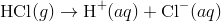A weak acid is an acid that ionizes only slightly in an aqueous solution. Acetic acid (found in vinegar) is a very common weak acid. Its ionization is shown below.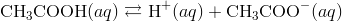The ionization of acetic acid is incomplete, and so the equation is shown with a double arrow. The extent of ionization of weak acids varies, but is generally less than 10%. A 0.10 M solution of acetic acid is only about 1.3% ionized, meaning that the equilibrium strongly favors the reactants.

Weak acids, like strong acids, ionize to yield the H + ion and a conjugate base. Because HCl is a strong acid, its conjugate base (Cl ) is extremely weak. The chloride ion is incapable of accepting the H + ion and becoming HCl again. In general, the stronger the acid, the weaker its conjugate base. Likewise, the weaker the acid, the stronger its conjugate base.

 Acid Conjugate Base Strong Acids HCl (hydrochloric acid) (strongest) Cl − (chloride ion) (weakest) H 2 SO 4 (sulfuric acid) HSO 4 − (hydrogen sulfate ion) HNO 3 (nitric acid) NO 3 − (nitrate ion) Weak Acids H 3 PO 4 (phosphoric acid) H 2 PO 4 − (dihydrogen phosphate ion) CH 3 COOH (acetic acid) CH 3 COO − (acetate ion) H 2 CO 3 (carbonic acid) HCO 3 − (hydrogen carbonate ion) HCN (hydrocyanic acid) (weakest) CN − (cyanide ion) (strongest)

Strong acids are 100% ionized in solution. Weak acids are only slightly ionized. Phosphoric acid is stronger than acetic acid and so is ionized to a greater extent. Acetic acid is stronger than carbonic acid, and so on.

#### The Acid Ionization Constant, Ka

The ionization for a general weak acid, HA, can be written as follows: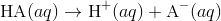Because the acid is weak, an equilibrium expression can be written. An acid ionization constant (Ka) is the equilibrium constant for the ionization of an acid.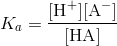The acid ionization represents the fraction of the original acid that has been ionized in solution. Therefore, the numerical value of Ka is a reflection of the strength of the acid. Weak acids with relatively higher Ka values are stronger than acids with relatively lower Ka values. Because strong acids are essentially 100% ionized, the concentration of the acid in the denominator is nearly zero and the Ka value approaches infinity. For this reason, Ka values are generally reported for weak acids only.

The Table below is a listing of acid ionization constants for several acids. Note that polyprotic acids have a distinct ionization constant for each ionization step, with each successive ionization constant being smaller than the previous one.

Acid Ionization Constants at 25°C
Name of Acid Ionization Equation Ka
Sulfuric acid

H2SO4 ⇌ H+ HSO4

HSO4 ⇌ H+ + SO42−

very large

1.3 × 10−2

Oxalic acid

H2C2O4 ⇌ H+ HC2O4

HC2O4 ⇌ H+ + C2O42−

6.5 × 10−2

6.1 × 10−5

Phosphoric acid

H3PO4 ⇌ H+ + H2PO4

H2PO4 ⇌ H+ HPO42−

HPO42− ⇌ H+ + PO43−

7.5 × 10−3

6.2 × 10−8

4.8 × 10−13

Hydrofluoric acid HF ⇌ H+ + F 7.1 × 10−4
Nitrous acid HNO2 ⇌ H+ + NO2 4.5 × 10−4
Benzoic acid C6H5COOH ⇌ H+ C6H5COO 6.5 × 10−5
Acetic acid CH3COOH ⇌ H+ + CH3COO 1.8 × 10−5
Carbonic acid

H2CO3 ⇌ H+ + HCO3

HCO3 ⇌ H+ + CO32−

4.2 × 10−7

4.8 × 10−11

Hydrocyanic acid HCN ⇌ H+ + CN 4.9 × 10−10

## Strong and Weak Bases and Base Ionization Constant (Kb)

### Ammonia for what?All the complex electronics and apparatus in a space shuttle generate heat, as do the astronauts. The shuttles have a complex arrangement of systems to dissipate that heat into outer space. One of the components of this system is a series of coils filled with ammonia that are located on the outside of the shuttle. Ammonia absorbs the heat and then releases it into space as the gas circulates through the coils. This approach is both inexpensive and effective.

### Strong and Weak Bases and Base Ionization Constant, Kb

As with acids, bases can either be strong or weak, depending on their extent of ionization. A strong base is a base, which ionizes completely in an aqueous solution. The most common strong bases are soluble metal hydroxide compounds such as potassium hydroxide. Some metal hydroxides are not as strong simply because they are not as soluble. Calcium hydroxide is only slightly soluble in water, but the portion that does dissolve also dissociates into ions.

A weak base is a base that ionizes only slightly in an aqueous solution. Recall that a base can be defined as a substance, which accepts a hydrogen ion from another substance. When a weak base such as ammonia is dissolved in water, it accepts an H + ion from water, forming the hydroxide ion and the conjugate acid of the base, the ammonium ion.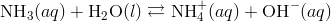The equilibrium greatly favors the reactants and the extent of ionization of the ammonia molecule is very small.

An equilibrium expression can be written for the reactions of weak bases with water. Because the concentration of water is extremely large and virtually constant, the water is not included in the expression. A base ionization constant (Kb) is the equilibrium constant for the ionization of a base. For ammonia the expression is: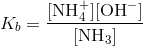The numerical value of Kb is a reflection of the strength of the base. Weak bases with relatively higher Kb values are stronger than bases with relatively lower Kb values. The Table below is a listing of base ionization constants for several weak bases.

Base Ionization Constants at 25°C
Name of Base Ionization Equation Kb
Methylamine CH3NH2 + H2O ⇌ CH3NH3+ + OH 5.6 × 10−4
Ammonia NH3 + H2O ⇌ NH4+ + OH 1.8 × 10−5
Pyridine C5H5N + H2O ⇌ C5H5NH+ + OH 1.7 × 10−9
Acetate ion CH3COO + H2O ⇌ CH3COOH + OH 5.6 × 10−10
Fluoride ion F + H2O ⇌ HF + OH 1.4 × 10−11
Urea H2NCONH2 + H2O ⇌ H2CONH3+ + OH 1.5 × 10−14

Notice that the conjugate base of a weak acid is also a strong base. For example, the acetate ion has a small tendency to accept a hydrogen ion from water to form acetic acid and the hydroxide ion.

## Calculating Ka and Kb

### Who invented the pH meter?

The pH meter was invented because Florida orange growers needed a way to test the acidity of their fruit. The first meter was invented by Arnold Beckman, who went on to form Beckman Instruments.

Beckman’s business was very successful and he used much of his fortune to fund science education and research. The Beckman family donated \$40 million to build the Beckman Institute at the University of Illinois.

### Calculating Ka and Kb

The numerical value of Ka or Kb can be determined from an experiment. A solution of known concentration is prepared and its pH is measured with an instrument called a pH meter .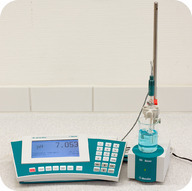Figure 1. A pH meter is a laboratory device that provides quick, accurate measurements of the pH of solutions.

#### Sample Problem: Calculation of an Acid Ionization Constant

A 0.500 M solution of formic acid is prepared and its pH is measured to be 2.04. Determine the Ka for formic acid.

Step 1: List the known values and plan the problem.

Known

• initial [HCOOH] = 0.500 M
• pH = 2.04

Unknown

• K= ?

First, the pH is used to calculate the [H + ] at equilibrium. An ICE table is set up in order to determine the concentrations of HCOOH and HCOO at equilibrium. All concentrations are then substituted into the Ka expression and the Ka value is calculated.

Step 2: Solve.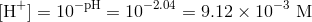Since each formic acid molecule that ionizes yields one H + ion and one formate ion (HCOO ), the concentrations of H + and HCOO are equal at equilibrium. We assume that the initial concentrations of each ion are zero, resulting in the following ICE table.

 Concentrations [HCOOH] [H + ] [HCOO − ] Initial 0.500 0 0 Change -9.12 × 10 -3 +9.12 × 10 -3 +9.12 × 10 -3 Equilibrium 0.491 9.12 × 10 -3 9.12 × 10 -3

Now substituting into the Ka expression gives: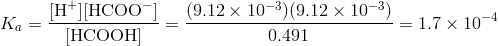The value of Ka is consistent with that of a weak acid. Two significant figures are appropriate for the answer, since there are two digits after the decimal point in the reported pH.

Similar steps can be taken to determine the Kb of a base. For example, a 0.750 M solution of the weak base ethylamine (C2H5 NH2) has a pH of 12.31.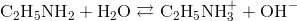Since one of the products of the ionization reaction is the hydroxide ion, we need to first find the [OH ] at equilibrium. The pOH is 14 – 12.31 = 1.69. The [OH ] is then found from 10 -1.69  = 2.04 × 10 -2  M. The ICE table is then set up as shown below.

 Concentrations [C2H5NH2] [C2H5NH3+] [OH−] Initial 0.750 0 0 Change -2.04 × 10 -2 +2.04 × 10 -2 +2.04 × 10 -2 Equilibrium 0.730 2.04 × 10 -2 2.04 × 10 -2

Substituting into the Kb expression yields the Kb for ethylamine.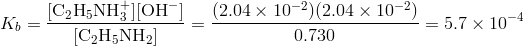### Summary

• Strong and weak acids are defined.
• The acid ionization constant (Ka) is defined.
• Strong and weak bases are defined.
• The base equilibrium constant is stated.
• Calculations of Ka and Kb are described.

### Practice

#### Strong and Weak Acids and Acid Ionization Constant (Ka)

http://www.chemguide.co.uk/physical/acidbaseeqia/acids.html

1. Why is the [H2O] factor not included in the expression for Ka ?
2. When we write H+, what are we really talking about?
3. Which is the weaker acid: methanoic or ethanoic acid?

#### Strong and Weak Bases and Base Ionization Constant (Kb)

http://www.chemguide.co.uk/physical/acidbaseeqia/bases.html

1. In the presence of hydroxide ions, which way does the equilibrium shift for the equation showing the dissociation of water?
2. What percentage of ammonia in solution actually produces hydroxide ions?
3. How does the position of equilibrium relate to the strength of a weak base?

#### Calculating Ka and Kb

http://www.ausetute.com.au/kb.html

1. What does an Arrhenius base dissociate to?
2. What does a Brønsted-Lowry base form in water?
3. How is percent ionization determined?

### Review

#### Strong and Weak Acids and Acid Ionization Constant (Ka)

1. Why is the chloride ion the weakest conjugate base?
2. What is the percent ionization in a 0.1 M solution of acetic acid?
3. Which is the stronger acid: HF or benzoic acid?

#### Strong and Weak Bases and Base Ionization Constant (Kb)

1. Define a strong base.
2. Define a weak base.
3. Which is the stronger base: pyridine or urea?

#### Calculating Ka and Kb

1. What approach is used for calculation of ionization constants?
2. What initial assumptions are made?
3. What equilibrium assumptions are made?

### Glossary

• acid ionization constant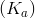: The equilibrium constant for the ionization of an acid.
• strong acid: An acid that is completely ionized in aqueous solution.
• weak acid: An acid that ionizes only slightly in aqueous solution.
• base ionization constant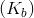: The equilibrium constant for the ionization of a base.
• strong base: A base which ionizes completely in aqueous solution.
• weak base: A base that ionizes only slightly in aqueous solution.
• pH meter: A device for accurate measurement of pH.